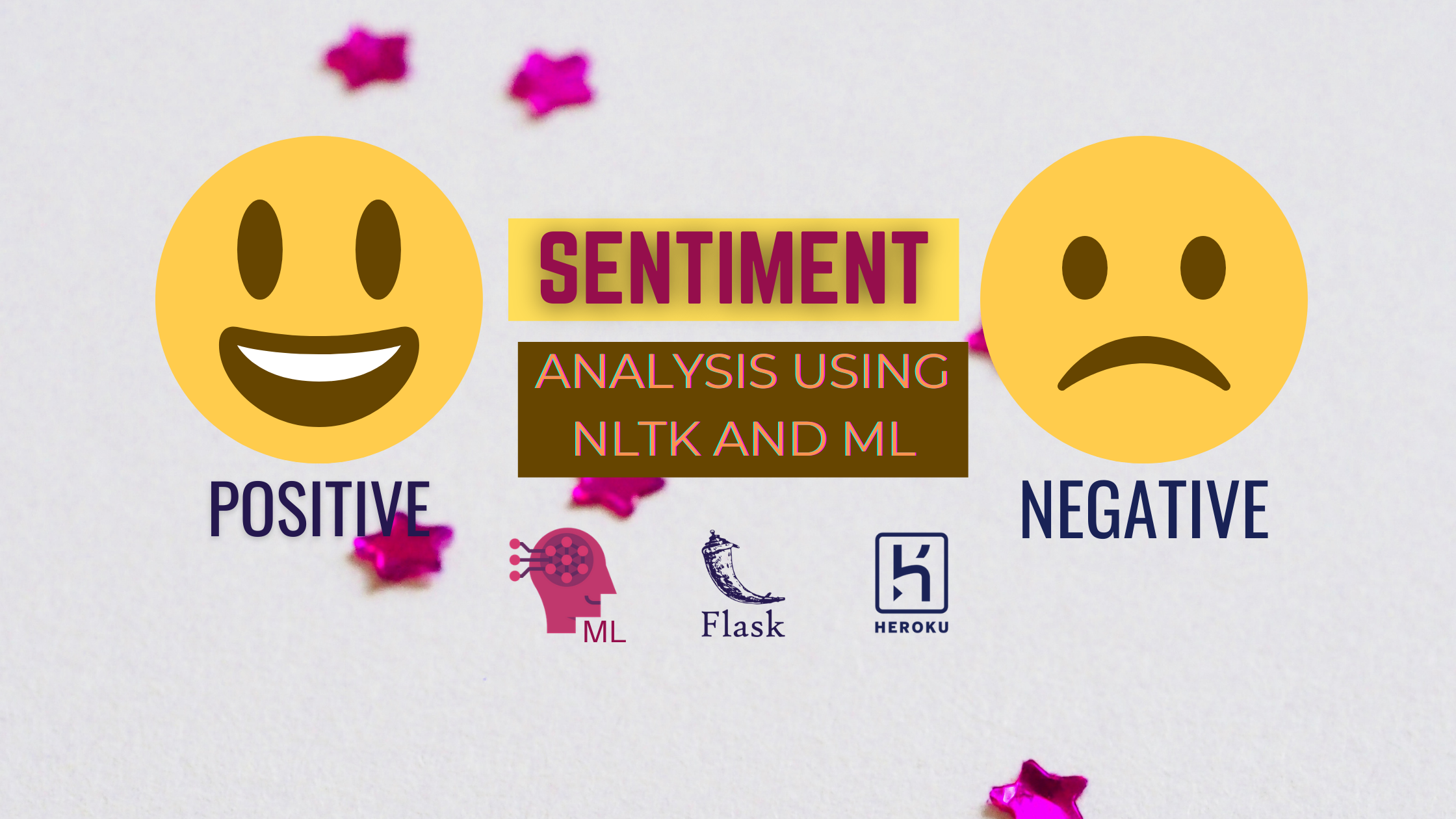# Sentiment Analysis web app using NLTK and Heroku# Creating a model using NLTK and ML

## Importing necessary libraries

`import numpy as np ## scientific computationimport pandas as pd ## loading dataset fileimport matplotlib.pyplot as plt ## Visulizationimport nltk  ## Preprocessing Reviewsnltk.download('stopwords') ##Downloading stopwordsfrom nltk.corpus import stopwords ## removing all the stop wordsfrom nltk.stem.porter import PorterStemmer ## stemming of wordsimport re  ## To use Regular expression`

NLTK: Natural Language Processing Toolkit is a python library that is used for performing all the NLP tasks like stemming, lemmatizing or removing stopwords, etc.

Porter Stemmer: It is a type of stemmer that is used for stemming. stemming is basically a technique of converting a word to its root word.
Ex: learning → learn, earning → earn

# Dataset

The dataset which we are going to use is an open source dataset available on kaggle.

The dataset is in the form of .tsv file. tsv means tab seorated file, to use this data we need to define a delimiter and quoting.

## Columns

Dataset has two columns Review and Liked.
Review: It contains the reviews of different custumers.
Liked: it is a numerical column. 0 means negative and 1 means positive review.

Our task is to create a machine learning model which can classify the sentiment for the upcoming review.

## Load the dataset in notebook

`dataset = pd.read_csv("Restaurant_Reviews.tsv",delimiter = "\t",quoting=3)`

at default the delimiter is set to `,` but because we are dealing with a tsv file so we need to change it to `\t` . Quoting is used to define whether the Quotes will be included or not. In generally we define the value of its as magic number 3.

# EDA on Dataset

`print(data.shape)  ### Return the shape of data print(data.ndim)   ### Return the n dimensions of dataprint(data.size)   ### Return the size of data print(data.isna().sum())  ### Returns the sum fo all na valuesprint(data.info())  ### Give concise summary of a DataFrameprint(df.head())  ## top 5 rows of the dataframeprint(df.tail()) ## bottom 5 rows of the dataframe`

After running the above code block you will see that we don’t have any null values in our dataset. Also, one thing to notice is that only one column of our has numerical values so we can only visualize that column.

Let’s visualize Liked Column

`import seaborn as snssns.countplot('Liked',data=dataset)`

Here in the above bar graph we can see that both our Positive and Negative sentiments are equal.

# Cleaning The Dataset

The most important step in any NLP problem is to clean the data and convert it into vector representation so that we can fed the data to a machine learning model.

`corpus = []for i in range(0,1000):   #we have 1000 reviews     review = re.sub('[^a-zA-Z]'," ",dataset["Review"][i])     review = review.lower()     review = review.split()     pe = PorterStemmer()     all_stopword = stopwords.words('english')     all_stopword.remove('not')     review = [pe.stem(word) for word in review if not word in set(all_stopword)]     review = " ".join(review)     corpus.append(review)print(corpus)`

## Explanation:

Line1: We define a empty list to store the cleaned version of reviews
Line2: A loop ranging from 0 to 1000 because we have only 1000 reviews
Line3: sub can replace anything in a sentence with anything. here we are replacing all the punctuation with a empty white space.
Line4: Converting the review to lower.
Line5: Spliting the review so that we have a list of words. we can also use word_tokenizer except this.
Line6: Creating a object for the class porterstemmer().
Line7: Initializing all the stopword in English dictionary to var all_stopwords.
Line8: Removing not from the stop words so that we can easily diffrenciate between positive and negative.
Line9: Running a loop in the length of the sentence and then for each word in the sentence checking it in stopword and if it does not find in stopword then apply Stemming on to the text and add it to the list.
Line10: Just concatenating all the words to make a sentence
Line11: appending the sentence to the corpus list
Line12: Printing the corpus list.

## Creating a Bage of words model for converting review into binary form

`from sklearn.feature_extraction.text import CountVectorizercv = CountVectorizer(max_features=1500) ##1500 columnsX = cv.fit_transform(corpus).toarray()y = dataset["Liked"]`

Line 1: We are importing the CountVectorizer from sklearn.
Line 2: Creating an object for the count vectorizer with max features as 1500, means we are only fetching the top 1500 columns.
Line 3: Using CV we are fitting are corpus and also transforming it into vectors and intilizing it to X.
Line4: Intilizing y with the values of column Liked.

## Dumping Counter Verctorizer object for future use

for doing the prediction for new reviews we need to dump cv and model both in a pkl file.

`import picklepickle.dump(cv, open('cv.pkl', 'wb'))`

# Modeling and Training

## Splitting the data into train and validation sets using train_test_split()

`from sklearn.model_selection import train_test_splitX_train,X_test,y_train,y_test = train_test_split(X,y,test_size=0.2,random_state=0)`

## Creating a model using MultinomialNB,GaussianNB

`from sklearn.naive_bayes import GaussianNB,MultinomialNBGNB = GaussianNB()MNB = MultinomialNB()`

## Fitting the X and y using both

`GNB.fit(X_train, y_train)MNB.fit(X_train, y_train)`

## Comparing both model based on accuracy on test data

`print(GNB.score(X_test,y_test))   ## 0.73print(MNB.score(X_test,y_test))   ## 0.775`

Here we can see that Multonial Naive Bayes with a accuracy of 77.5% is highest.

## Prediction

`y_pred=model.predict(X_test)print(np.concatenate((y_pred.reshape(len(y_pred),1), np.array(y_test).reshape(len(y_test),1)),1))`

# Evaluating Model

we are going to evaluate our model using the confusion matrix and accuracy score.

`from sklearn.metrics import confusion_matrix,accuracy_scorecm = confusion_matrix(y_test, y_pred)score = accuracy_score(y_test,y_pred)print(cm,score*100)`

Output:

`[[74 23]   [22 81]]  77.5`

We are going to save our model using pickle same as before.

`import pickle# Save trained model to filepickle.dump(cls, open("review.pkl", "wb"))`

`loaded_model = pickle.load(open("review.pkl", "rb"))y_pred_new = loaded_model.predict(X_test)loaded_model.score(X_test,y_test)`

Revaluate our model

`from sklearn.metrics import confusion_matrix,accuracy_scorecm = confusion_matrix(y_test,y_pred_new)score = accuracy_score(y_test,y_pred_new)print(cm,score*100)`

Output:

`[[74 23]   [22 81]]  77.5`

# Predicting Sentiment For a New Review

`def new_review(new_review):  new_review = new_review  new_review = re.sub('[^a-zA-Z]', ' ', new_review)  new_review = new_review.lower()  new_review = new_review.split()  ps = PorterStemmer()  all_stopwords = stopwords.words('english')  all_stopwords.remove('not')  new_review = [ps.stem(word) for word in new_review if not word in  set(all_stopwords)]  new_review = ' '.join(new_review)  new_corpus = [new_review]  new_X_test = cv.transform(new_corpus).toarray()  print(new_X_test.shape)  new_y_pred = loaded_model.predict(new_X_test)  return new_y_prednew_review = new_review(str(input("Enter new review...")))if new_review==1:   print("Positive")else :   print("Negative")`